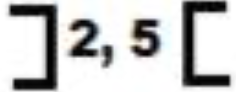Interval Notation is a method to denote that all numbers that lie between two other numbers belong to a set, with or without including the two numbers. The two numbers are called the “endpoints” of the interval.

Question: How to represent all the real numbers that lie between 2 and 5?
Answer: the interval notation is the method or the set that will denote the existence of all real numbers between 2 and 5, including or excluding 2 and 5 as the case may be.

But how? As follows: The Interval notation, which is actually a set uses either Brackets or Parentheses. The Bracket symbol [ ] is used for inclusive counting, while the Parentheses symbol ( ) is used for exclusive counting.

Brackets [ ]

The Interval notation making use of Brackets is used for inclusive counting, i.e. for counting all the numbers between the two endpoints, including the two endpoints.

Example

All real numbers between 2 and 5, including 2 and 5 is denoted as [2, 5]
The interval [2, 5] making use of the brackets [ ] denotes the set containing all the real numbers that lie between 2 and 5, including the two endpoints 2 and 5.

Parentheses: ( )

Interval notation making use of Parentheses is for exclusive counting, i.e. for counting only numbers that lie between the two endpoints, excluding the two endpoints.

Example

All real numbers lying between 2 and 5, excluding 2 and 5 is denoted by the interval (2, 5). The interval (2, 5) using parentheses ( ) denotes the set containing only the real numbers that lie between 2 and 5, without including the two endpoints 2 and 5. Important note about interval notation using parentheses: For parentheses type of interval notation, brackets may also be used, but they must be written inverted. For example the interval (2, 5) can also be denoted with inverted square brackets as below:## 1. Closed interval:

The interval notation [2, 5] is called closed interval for expressing the fact or property that the set (i.e. the interval) contains all real numbers from 2 to 5, including the end points 2 and 5.

## 2. Open Interval:

The interval notation (2, 5) is called open interval for expressing the fact or property that the set (i.e. the interval) contains all real numbers from 2 to 5, excluding the end points 2 and 5.

Other possible types of interval notation:

## 3. Left Closed Right Open Interval notation: [ )

For example: [2, 5) is an interval or set that contains all real numbers between 2 and 5, including 2 and excluding 5.

## 4. Left open, right closed interval notation ( ]

For example (2, 5] is an interval or set that contains all real numbers between 2 and 5, excluding 2 and including 5. Interval Notation with Infinity:

Since Infinity is not a number, but only a concept, therefore, only open interval can be written for it.

1. With positive infinity – the interval notation for all real numbers greater than or equal to 2 is [2, ∞)

2. With negative infinity – the interval notation for all real numbers less than or equal to 2 is (- ∞, 2]